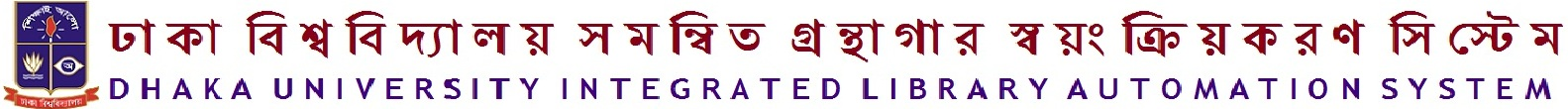Normal view MARC view ISBD view

# A conceptual guide to statistics using SPSS /

Material type:BookPublisher: Los Angeles : Sage, c2012Description: xiii, 296 p. : ill. ; 24 cm.ISBN: 9781412974066 (pbk. : alk. paper).
Contents:
Machine generated contents note: 1. Introduction 2. Descriptive Statistics 3. Chi-Squared Test 4. Linear Correlation 5. One- and Two Sample T-Tests 6. One-way ANOVA 7. Two- and Higher-way ANOVA 8. Within-subject ANOVA 9. Mixed-model ANOVA 10. MANOVA 11. Regression 12. ANCOVA 13. Factor and Components Analysis 14. Psychometrics 15. Non-parametric Tests 16. Matrix Algebra 17. Appendix on the General Formulation of Custom Contrasts using Syntax .
Summary: "This book helps students develop a conceptual understanding of a variety of statistical tests by linking the statistics with the computational steps and output from SPSS. Learning how statistical ideas map onto computation in SPSS will help students build a better understanding of both. For example, seeing exactly how the concept of variance is used in SPSS-how it is converted into a number based on real data, which other concepts it is associated with, and where it appears in various statistical tests-will not only help students understand how to use statistical tests in SPSS and how to interpret their output, but will also teach them about the concept of variance itself. Each chapter begins with a student-friendly explanation of the concept behind each statistical test and how the test relates to that concept. The authors then walk through the steps to compute the test in SPSS and the output, pointing out wherever possible how the SPSS procedure and output connects back to the conceptual underpinnings of the test. Each of the steps is accompanied by annotated screen shots from SPSS, and relevant components of output are highlighted in both the text and in the figures. Sections explain the conceptual machinery underlying the statistical tests. In contrast to merely presenting the equations for computing the statistic, these sections describe the idea behind each test in plain language and help students make the connection between the ideas and SPSS procedures. These include extensive treatment of custom hypothesis testing in ANOVA, MANOVA, ANCOVA, and regression, and an entire chapter on the advanced matrix algebra functions available only through syntax in SPSS. The book will be appropriate for both advanced undergraduate and graduate level courses in statistics"-- Provided by publisher.
Tags from this library: No tags from this library for this title. Add tag(s)
Item type Current location Collection Call number Status Date due BarcodeBooks Dhaka University Science Library
General Stacks
Non Fiction 005.55 BEC (Browse shelf) Available 475019

Includes bibliographical references and indexes.

Machine generated contents note: 1. Introduction 2. Descriptive Statistics 3. Chi-Squared Test 4. Linear Correlation 5. One- and Two Sample T-Tests 6. One-way ANOVA 7. Two- and Higher-way ANOVA 8. Within-subject ANOVA 9. Mixed-model ANOVA 10. MANOVA 11. Regression 12. ANCOVA 13. Factor and Components Analysis 14. Psychometrics 15. Non-parametric Tests 16. Matrix Algebra 17. Appendix on the General Formulation of Custom Contrasts using Syntax .

"This book helps students develop a conceptual understanding of a variety of statistical tests by linking the statistics with the computational steps and output from SPSS. Learning how statistical ideas map onto computation in SPSS will help students build a better understanding of both. For example, seeing exactly how the concept of variance is used in SPSS-how it is converted into a number based on real data, which other concepts it is associated with, and where it appears in various statistical tests-will not only help students understand how to use statistical tests in SPSS and how to interpret their output, but will also teach them about the concept of variance itself. Each chapter begins with a student-friendly explanation of the concept behind each statistical test and how the test relates to that concept. The authors then walk through the steps to compute the test in SPSS and the output, pointing out wherever possible how the SPSS procedure and output connects back to the conceptual underpinnings of the test. Each of the steps is accompanied by annotated screen shots from SPSS, and relevant components of output are highlighted in both the text and in the figures. Sections explain the conceptual machinery underlying the statistical tests. In contrast to merely presenting the equations for computing the statistic, these sections describe the idea behind each test in plain language and help students make the connection between the ideas and SPSS procedures. These include extensive treatment of custom hypothesis testing in ANOVA, MANOVA, ANCOVA, and regression, and an entire chapter on the advanced matrix algebra functions available only through syntax in SPSS. The book will be appropriate for both advanced undergraduate and graduate level courses in statistics"-- Provided by publisher.

There are no comments for this item.